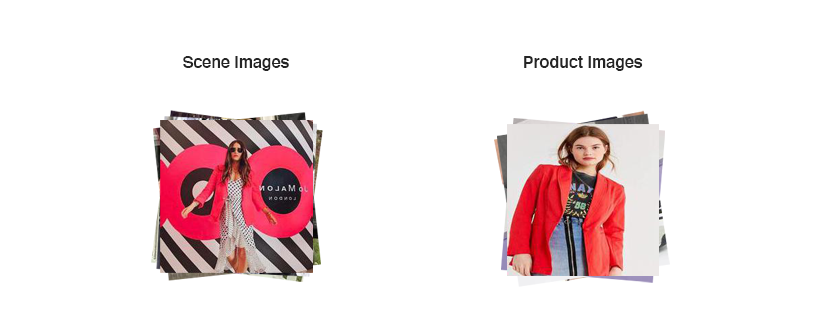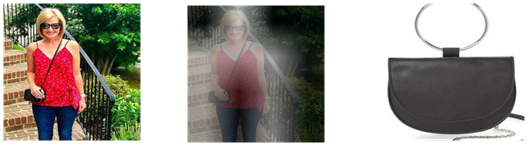Home > Blog > Artificial Intelligence > Secret Ingredients Of Pinterest’s Recommendations – Part 2

# Secret Ingredients Of Pinterest’s Recommendations – Part 2557rfq4th6

Aug 03, 2022

An effort of reimplementing Pinterest’s recommender system.

## Overview

In my last post about Complete The Look, I tried to explain what Pinterest did with their fashion recommender system. If you did not see this post, you could follow this link to get the main ideas to accomplish this task.

Complete The Look is a promising approach in the attempt to overcome the limitation of traditional fashion recommender systems. The old-fashioned systems often use images of products on a plain white background, whereas what the customers want to see is the way these products complement each other in daily scenes such as in street photos, travel lookbooks, and selfies.

This challenge makes Pinterest seek a solution to measure the compatibility between the products and the scenes, which is the core idea of Complete The Look.

When reaching the end of this tutorial, I hope you could understand:

• How the Complete The Look dataset is organized and processed
• How to reimplement this recommender system

The original paper could be found on Arxiv.

## Dataset Explanation

Before diving into the system, let’s explore the data.

Currently, there is no available dataset on the Internet for Complete The Look task. Pinterest team used the dataset of the similar task called Shop The Look to implement the system. We could find the dataset from this Github repository.

There are lots of things to talk about this dataset, but I will focus on important points.

We can see several JSON files on the repository. The two files fashion-cat.json and fashion.json contain all we need. The product signatures and its categories are in fashion-cat.json, and fashion.json give the signatures of the scene-product pair.

Later, we will mentioned to category images, in fact, these images are the product image in the scene-product pair.Example of a scene-product image pair

To generate a dataset for Complete The Look, the authors suggest to crop the scene images so that they could exclude the correct products.

For the sake of convenience, I already processed the data and publish it here. You just need to download this. The dataset I published is a set of scene-positive image-negative image triples, which is ready for the training in the next part.

## Fashion Recommender System

Complete The Look requires two inputs from users.

First, we should provide the scene image, in which we appear wearing some items that need to find other products to complement them. These images could be anything we posted on social networks. Next, the category is important for the system to know which products meet our needs. The most common categories are shirt, pants, and shoes.Fashion Recommender System with Complete The Look

The recommender system a two major parts. The Style Embedding is a neural network that has the responsibility to measure how well each product in the category could go with the scene image.

Based on the compatibility distance given by the Style Embedding, the Product Ranking part arrange the products in ascending order. The recommend products are on top of this ranking.

## Style Embedding

Style Embedding is a neural network that try to learn a good way to represent the scene and the products in the same space. Pinterest get image features from ResNet50 intermediate layers, then pass these features to some feed forward networks. The intermediate layers I chose are avg_pool and conv4_block6_out.

This is how I get the features from ResNet50. The features should not be trainable.

``class StyleEmbedding(object):\n    def __init__(self):\n        self._num_crop = 4\n        self.model = keras.applications.ResNet50(weights='imagenet', \ninput_shape=(224, 224, 3))\n        self.model.trainable = False\n        self.avg_pool = self.model.get_layer('avg_pool').output\n        self.conv4_6 = self.model.get_layer('conv4_block6_out').output``

Based on the original paper, we may need at least 3 new feed forward networks.

``class StyleEmbedding(object):\n\n# code\n\n   def build_g_model(self):\n       x = keras.layers.Dense(units=512)(self.avg_pool)\n       x = keras.layers.BatchNormalization()(x)\n       x = keras.layers.Activation('relu')(x)\n       x = keras.layers.Dropout(rate=0.1)(x)\n       x = keras.layers.Dense(units=128)(x)\n       x = keras.layers.Lambda(lambda x: tf.math.l2_normalize(x,axis=-1))(x)\n       \n       return keras.Model(inputs=self.model.inputs, outputs=x, name='g_model')\n\n   def build_l_model(self):      \n       x = keras.layers.Flatten()(self.conv4_6)\n       x = keras.layers.Dense(units=256)(x)\n       x = keras.layers.BatchNormalization()(x)\n       x = keras.layers.Activation('relu')(x)\n       x = keras.layers.Dropout(rate=0.1)(x)\n       x = keras.layers.Dense(units=128)(x)\n       x = keras.layers.Lambda(lambda x: tf.math.l2_normalize(x,axis=-1))(x)\n\n       return keras.Model(inputs=self.model.inputs, outputs=x, name='local_model_1')\n\n   def build_lh_model(self):\n       x = keras.layers.Flatten()(self.conv4_6)\n       x = keras.layers.Dense(units=128)(x)\n       x = keras.layers.BatchNormalization()(x)\n       x = keras.layers.Activation('relu')(x)\n       x = keras.layers.Dropout(rate=0.1)(x)\n       x = keras.layers.Dense(units=128)(x)\n       x = keras.layers.Lambda(lambda x: tf.math.l2_normalize(x,axis=-1))(x)\n\n       return keras.Model(inputs=self.model.inputs, outputs=x, name='local_model_2')``

To measure the compatibility, there are several types of distances we need to care about: global distance, local distance and hybrid distance.

## Global Distance

The global distance is simply the difference between the scene image and the product image.

To compute this distance, we need to implement the global distance layer.

``class GlobalDistanceLayer(keras.layers.Layer):\n    def __init__(self):\n        super(GlobalDistanceLayer,self).__init__()\n      \n    def call(self, inputs):\n        n = tf.norm(inputs-inputs, axis=-1)\n        n = tf.math.square(n)\n        return n``

## Local Distance

The local distance is an attention-based metric to measure the compatibility.

We will the cropping layer to crop the scene into some local regions.

``class CroppingLayer(keras.layers.Layer):\n    def __init__(self, offset_height, offset_width, target_height, target_width, size=[224,224]):\n        super(CroppingLayer,self).__init__()\n        self.trainable = False\n        self._offset_height = offset_height\n        self._offset_width = offset_width\n        self._target_height = target_height\n        self._target_width = target_width\n        self._size = size    \n\n\tdef call(self, inputs):\n        cropped = tf.image.crop_to_bounding_box(inputs, \n        \t\t\toffset_height=self._offset_height, \n        \t\t\toffset_width=self._offset_width, \n        \t\t\ttarget_height=self._target_height, \n        \t\t\ttarget_width=self._target_height)\n        return tf.image.resize(cropped, size=self._size)``

The attention weights help the system focus on where the items is likely to appear. These weights are computed by computing the difference of the category image and each region of the scene image. Then we need to scale the weights to range (0,1)(0,1) by the softmax function.

The local distance is the weighted sum of the difference between the product image and each region of the scene.

``class AttentionLayer(keras.layers.Layer):\n      def __init__(self):\n            super(AttentionLayer,self).__init__()\n\n      def call(self, inputs):\n            a = tf.math.reduce_euclidean_norm(inputs-inputs[tf.newaxis],axis=-1)\n            a = tf.math.square(a)\n            a = tf.nn.softmax(a,axis=0)\n            return a\n\nclass LocalDistanceLayer(keras.layers.Layer):\n      def __init__(self):\n            super(LocalDistanceLayer,self).__init__()\n\n      def call(self, inputs):\n            d = tf.norm(inputs-inputs[tf.newaxis],axis=-1)\n            d = tf.math.square(d)\n            d = tf.math.multiply(d,inputs)\n            d = tf.math.reduce_sum(d,axis=0)\n\n            return d``

Here are some results of our attention weights.

## Hybrid Distance

The compatibility should depend on how the products could with the local regions and the entire scene. Therefore, the hybrid distance is the mean of the global distance and the local distance.

Here is the implementation of the hybrid distance layer.

``class HybridDistanceLayer(keras.layers.Layer):\n    def __init__(self,name=None):\n        super(HybridDistanceLayer,self).__init__(name=name)    \n    def call(self,inputs):\n         d = 0.5*(inputs+inputs)\n         return d``

It’s time to build the final model.

``class StyleEmbedding(object):\n\tdef __call__(self):\n       scene_inputs = keras.Input((224,224,3),name='scene_input')\n       pl_inputs = keras.Input((224,224,3),name='positive_input')\n       mn_inputs = keras.Input((224,224,3),name='negative_input')\n       \n       g_model = self.build_g_model()\n       lh_model = self.build_lh_model()\n       l_model = self.build_l_model()\n\n       fs = g_model(scene_inputs)\n       fpp = g_model(pl_inputs)\n       fpm = g_model(mn_inputs)\n       c = lh_model(pl_inputs)\n      \n  \n       regions = []\n       step = 224//self._num_crop\n       for i in range(self._num_crop):\n           for j in range(self._num_crop):\n               regions.append(CroppingLayer(offset_height=i*step, offset_width=j*step, target_height=step, target_width=step)(scene_inputs))\n       \n       fis = []\n       fihs = []\n       for i in range(self._num_crop*self._num_crop):\n           fis.append(l_model(regions[i]))\n           fihs.append(lh_model(regions[i]))\n       fis = tf.stack(fis)\n       fihs = tf.stack(fihs)\n       \n       a = AttentionLayer()([fihs,c])\n       pld = LocalDistanceLayer()([fis,fpp,a])\n       mld = LocalDistanceLayer()([fis,fpm,a])\n       pgd = GlobalDistanceLayer()([fs,fpp])\n       mgd = GlobalDistanceLayer()([fs,fpm])\n       pd = HybridDistanceLayer(name='y_positive')([pgd,pld])\n       md = HybridDistanceLayer(name='y_negative')([mgd,mld])\n       outputs = tf.stack([pd,md])\n  \n       return keras.Model(inputs=[scene_inputs,pl_inputs,mn_inputs], outputs=outputs)``

## Triplet loss

Complete The Look uses the triplet loss to train the model. Triplet loss is a common loss function in machine learning that takes 3 inputs. These are the correct product, the compatible product, and the incompatible product.

``@tf.function\ndef compat_loss(y_true,y_pred):\n    x = y_pred - y_pred + 0.2\n    x = tf.math.maximum(x,0.0)\n    x = tf.math.reduce_sum(x)\n    return x``

## Product Ranking

After achieve the compatibility distance, product ranking show us which product is the most compatible to the scene.

Here is how I try to implement the product ranking.

``index = 76 # image index in the test set\n\nscene = data['scene'][index].numpy()\npositive = data['positive'][index].numpy()\nnegative = data['negative'][index].numpy()\ncategory_label = data['category'][index]\nscene_input = keras.applications.resnet.preprocess_input(scene.reshape((1,224,224,3)))\npositive_input = keras.applications.resnet.preprocess_input(positive.reshape((1,224,224,3)))\nnegative_input = keras.applications.resnet.preprocess_input(negative.reshape((1,224,224,3)))\n\nsign_idx = np.where(np.array(data['category']) == category_label)\nsign = np.unique(np.array(data['positive_sign'])[sign_idx])\nproduct_idx = []\n\nfor i in range(len(sign)):\n    product_idx.append(np.where(np.array(data['positive_sign'])==sign[i]))\nproduct_idx = np.array(product_idx)\nproducts =  np.array(data['positive'])[product_idx]\nproduct_inputs = keras.applications.resnet.preprocess_input(products)\nscene_inputs = np.array(tf.repeat(scene_input,repeats=len(product_idx),axis=0))\nnegative_inputs = np.array(tf.repeat(negative_input,repeats=len(product_idx),axis=0))\npred = distance_model.predict([scene_inputs,product_inputs,negative_inputs],batch_size=1)\n\ntop_idx = np.argsort(pred)``

Let’s see how well our recommender system is.The scene image and the ground truth